# How to linearize max, min, and abs functions

In this post you will see how to linearize max functions, min functions, and absolute value functions. These can be expressed mathematically as: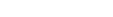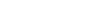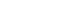Let’s start with the modulus, as the other two depend on it.Here you simply replace that one constraint by the following two: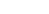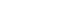The min function can be linearized as:First, we decompose the min function into a max and a modulus.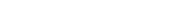Then we get rid of the max.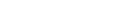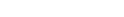Finally, the answer is using the following five constraints: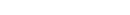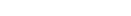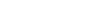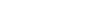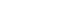Remember to penalize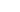and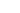such that only one of them will be different from zero in any solution.

The max function can be linearized as follows: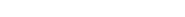From here on things are very similar to the min function…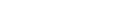And finally, the five necessary constraints are:And again, remember to penalizeand.

Thanks to Lieke van der Heide.

This was useful? Buy me a cup of coffee to help me keep this website running!